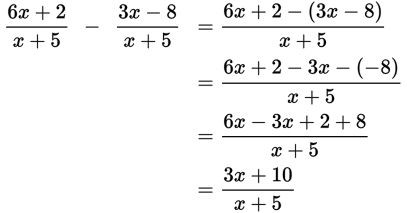# SAT Math Multiple Choice Question 351: Answer and Explanation

### Test Information

Question: 351

6.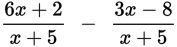Which of the following is equivalent to the expression above?

• A.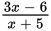• B.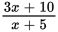• C.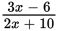• D.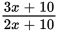Explanation:

B

Difficulty: Medium

Category: Passport to Advanced Math / Exponents

Strategic Advice: When the denominators of rational expressions are the same, you can combine the numerators and keep the same denominator.

Getting to the Answer: The terms in the expression have the same denominator, x + 5, so their numerators can be subtracted. Simply combine like terms and keep the denominator the same. Don't forget to distribute the negative to both 3x and -8.